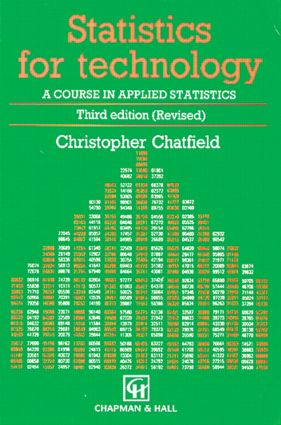Statistics for Technology

A Course in Applied Statistics, Third Edition, 3rd Edition

Chapman and Hall/CRC

384 pages

Purchasing Options:\$ = USD
Paperback: 9780412253409
pub: 1983-06-01
SAVE ~\$20.00
\$100.00
\$80.00
x
Hardback: 9781138469877
pub: 2017-09-28
SAVE ~\$41.00
\$205.00
\$164.00
x
eBook (VitalSource) : 9780203738467
pub: 2018-02-19
from \$48.48

FREE Standard Shipping!

Description

One of the most popular introductory texts in its field, Statistics for Technology: A Course in Applied Studies presents the range of statistical methods commonly used in science, social science, and engineering.

The mathematics are simple and straightforward; statistical concepts are explained carefully; and real-life (rather than contrived) examples are used throughout the chapters.

Divided into three parts, the Introduction describes some simple methods of summarizing data. Theory examines the basic concepts and theory of statistics. Applications covers the planning and procedures of experiments, quality control, and life testing.

Revised throughout, this Third Edition places a higher priority on the role of computers in analysis, and many new references have been incorporated. A new appendix describes general methods of tackling statistical problems, including guidance on literature searching and report writing.

Reviews

"The book will appeal not only to technologists requiring an introduction to statistics, but to lecturers in applied statistics and those running statistical 'service' courses for other disciplines. The industrial statistician will also find material useful in his dealings with engineers and technologists."

-Journal of the Royal Statistical Society

Promo Copy

INTRODUCTION

Outline of Statistics

Simple Ways of Summarizing Data

Introduction

Graphical Methods

Summary Statistics

THEORY

The Concept of Probability

Probability and Statistics

Some Definitions

Types of Events

Permutations and Combinations

Random Variables

Discrete Distributions

The Discrete Probability Distribution

The Binomial Distribution

The Binomial Model

Types of Distribution

The Mean and Variance of the Binomial Distribution

The Poisson Distribution

Bivariate Discrete Distributions

Continuous Distributions

Definitions

The Mean and Variance of Continuous Distributions

The Normal Distribution

Uses of the Normal Distribution

Normal Probability Paper

The Exponential Distribution

Bivariate Continuous Distributions

Estimation

Point and Interval Estimates

Properties of the Expected Value

The Sampling Distribution of (INSERT SYMBOL)

The Sampling Distribution of s2

Some Properties of Estimators

General Methods of Point Estimation

Interval Estimation

Significance Tests

Introduction

Tests on a Sample Mean

Comparing Two Sample Means

The t- Test Applied to Paired Comparisons

The x2 Goodness-of-Fit Test

The F- test

Distribution-Free or Non-Parametric Tests

Power and Other Considerations

Regression and Correlation

Scatter Diagram

Curve Fitting

Regression

Confidence Intervals and Significance Tests in Linear Regression

The Coefficient of Determination

Multiple and Curvilinear Regression

Orthogonal Polynomials

The Design of Regression Experiments

The Correlation Coefficient

Estimating the Regression Lines

The Bivariate Normal Distribution

Interpretation of the Correlation Coefficient

APPLICATIONS

Planning the Experiment

Preliminary Remarks

Measurements

The Propagation of Error

Improving Precision With Series and Parallel Arrangements

Combining Dissimilar Estimates by the Method of Least Squares

The Design and Analysis of Experiments-1: Comparative Experiments

Some Basic Considerations in Experimental Design

A Mathematical Model for Simple Comparative Experiments

The Number of Replications

Randomization

The Analysis of a Randomized Comparative Experiment

The Range Test

One-Way Analysis of Variance

Follow-Up Study of the Treatment Means

Verifying the Model

The Randomized Block Experiment

Two-Way Analysis of Variance

Latin Squares

Balanced Incomplete Block Designs

The Design and Analysis of Experiments-2: Factoral Experiments

Introduction

The Advantages of Complete Factorial Experiments

The Design of Complete Factorial Experiments

The Analysis of a Complete Factorial Experiment

Follow-Up Procedure

The 2n Factorial Design

Fixed Effects and Random Effects

Other Topics

The Examination of Residuals

Determination of Optimum Conditions

Summary

Quality Control

Acceptance Sampling

Operating Characteristic Curve

Types of Sampling Schemes

Rectifying Schemes

The Military Standard Plan

Sampling by Variables

Practical Problems

Process Control

Control Charts for Samples

Cusum Charts

Prediction, System Identification, and Control

Life Testing

Problems in Measuring Reliability

The Mathematical Distribution of Failure Times

The Exponential Distribution

Estimating the Conditional Failure Rate

The Weibull Distribution

Appendix A: The Relationships Between the Normal X2, t- and F-distributions

Appendix B: Statistical Tables

Table 1. Areas Under the Normal Curve

Table 2. Percentage Points of Student's t- Distribution

Table 3. Percentage Points of the x2 Distribution

Table 4. Upper Percentage Points of the F- Distribution

Table 5. Values of e-x

Table 6. Percentage Points of the Distribution of the Studentized Range

Table 7. Random Numbers

Appendix C: Further Reading

Appendix D: Some Other Topics

Calculating the Mean and Standard Deviation of a Frequency Distribution

Interpretation of the Sample Standard Deviation

How to Round Numbers

Stem-and-Leaf Plots and Box Plots

Estimating and Testing a Proportion

Appendix E: Some General Comments on Tackling Statistical Problems

Preliminary Questions

Collecting the Data

Preliminary Data Analysis

Choice of a More Elaborate Method

Using a Computer

Using a Library

Presenting the Results